# Strengthening Techniques of Reinforced Concrete Beams-Bond CharacteristicsReading time: 1 minute

Strengthening of reinforced concrete beam utilizing FRP has been applied to tackle problems related to the civil infrastructure. There are various studies which demonstrate the effectiveness of different strength techniques using FRP and considerably increase both strength and stiffness of concrete element. Brittle failure of strengthened concrete beam because of FRP delamination is likely which may restrict the application of FRP full potential. Therefore, studying and understanding the bond between FRP and concrete is crucial in order to improve concrete beams appropriately. Characteristics of the bond between concrete and FRP bars, strips, and sheets in different strengthening technique for example near surface mounted and externally bonded technique will be discussed in the following sections.

## Bond between Concrete and FRP Bars in NSM Technique

The most important factor that controls the performance of concrete elements improved by the NSM FRP bar is the bond between concrete and FRP bars so proper understanding of bond behavior is substantially significant for enhancing the reinforced concrete beams successfully. Bar-resin and resin-concrete are the two interfaces included in the NSM FRP. Tensile stress is generated in the FRP reinforcement after loading of the structure. The resultant stress imposed by the lug of FRP bar on the adhesive material is acts at an angle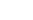to the line of the longitudinal bar as illustrated in Figure-1 and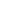is the coefficient of friction which is equal to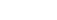.Fig.1: Forces Between NSM FRP Bar and Epoxy

Moreover, at both concrete-adhesive and adhesive-FRP bar interfaces; the radial component of the resultant stress generates regions of high tensile stresses. The distribution of stresses around FRP bars are shown in Figure-2. As it can be observed from the figure, there is discontinuity of stresses at concrete epoxy interface; this is due to the difference between elastic modulus of concrete and epoxy.Fig.2: Typical Distribution of Stress Around FRP Bars

There are three mechanisms that oppose the stress that produced due to loading of the concrete element. Firstly, resistance of the deformed FRP bar during interlocking with the concrete surface, which is called mechanical interlocking. Secondly, chemical bonding of both concrete and bar interfaces (adhesion) and, finally, the friction resistance between concrete and FRP bar interfaces. These mechanisms are dependent on the surface configuration of the bars. For example, all kind of resistances will be present if the bar has a deformed surface and adhesion is dominant at the beginning of loading, and after the failure of the chemical bond, the friction resistance mechanism will be activated. When the surface of the bar is smooth, only adhesion and friction withstand the longitudinal shear stress. The surface of FRP bars is covered with resin, so the strength of resin influences the bond considerably. Epoxy split failure and concrete split failure are two different modes of failure that occur in a strengthened reinforced concrete beam. The latter happens when the tensile strength of concrete is exceeded by the tensile stress in the concrete-epoxy interface. This tensile stress can be reduced by increasing the width of groove cuttings. Utilizing a binder material that possesses high tensile strength and/or raising the thickness of epoxy material could delay epoxy split failure and consequently concrete split failure will occur. The tangential bond stress (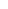) in the strengthened concrete beam can be computed from the following equation: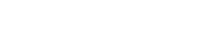Where: d: bar diameter fFRP: Stress in the NSM FRP bar Ld: Embedment length required to develop stress in the NSM FRP bar And the radial stress can be calculated as follow: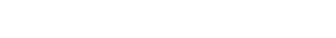The tensile stress at concrete-epoxy (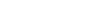) interface can be found as: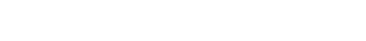Furthermore, the tensile stress at FRP-epoxy (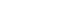) is estimated by the following equation and based on G2 or G’2 whichever provide larger value: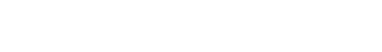Where: G1, G2, and G’2: Coefficients determined from finite element analysis All stresses which are mentioned above are shown in Figure-2. Finally, the embedment length required to avoid concrete split failure can be expressed as: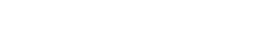And embedment length needed to prevent epoxy split failure is: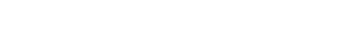Where, fct and fepoxy: are the concrete and epoxy tensile strength, respectively

## Bond between NSM FRP Strip and Concrete

Since NSM FRP strips have two sides, so there is two bonded side area between FRP strip and concrete. An analytical model has been developed to estimate the interfacial shear stress of the FRP strip. Not only does the model taken the two-side bonded area into consideration but also the continuous declining of flexural stiffness because of concrete cracking. It is considered that large concentration of shear stresses at the end of the strips cause the strip debonding. When simply supported beam subjected to point load (P) at the midspan, the shear stress at the point where FRP strip is terminated can be evaluated as follow:Where:: is the shear stress at the end of the NSM FRP strips. tf: is the thickness of the NSM FRP strips. n: is the modular ratio between concrete FRP and concrete. P: is the concentrated failure load. lo: is the unbonded length of the strip yeff: is the distance from the neutral axis of the beam to the NSM FRP strips. Ieff: is the effective moment of inertia of enhanced element. x: is the distance between the end of the NSM FRP strip to the beam support. Ga: is the shear modulus of the binder agent. ta: is the thickness of the adhesive materials Ef: is the elastic modulus of the FRP strips. When the shear stress, which depends on the concrete properties, reaches the maximum value, debonding will take place. It is demonstrated that, the failure of strengthened beams is started and controlled by concrete shear strength. Moreover, strength and adhesion properties of other kinds of materials such as epoxy, and NSM FRP strip are much stronger than concrete. Furthermore, the ultimate shear stress in the strengthened beam can be computed if both compressive and tensile strength of concrete are known by applying the following equation.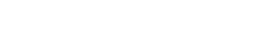Where fc': Compressive strength of concrete after 28 days fct: Tensile strength of concrete The magnitude of the load that causes the failure of strengthened beam can be found by substituting equation 9 into equation 7. Regarding development length, it relies on FRP strip dimensions, concrete properties, adhesive properties, internal steel reinforcement ratio, reinforcement arrangement, type of loading, and groove width. The development length of FRP strip can be calculated by considering these steps. Firstly, calculate loading failure for various embedment lengths, consequently a curve which represent failure envelope because of strip debonding at cut off point is produced as shown in Figure-3. Secondly, apply a cracked section analysis at sections of ultimate induced normal stresses and calculate maximum load need to rupture the strip as shown in Figure-3. Thirdly, find the development length at the intersection of the line corresponds to the strip flexural failure with curve representing debonding failure at the end of the strip. Finally, it should be said that, development length will prevent brittle failure due to strip debonding and guarantee complete composite action between concrete and FRP strip.Fig.3: Procedure to Estimate Development Length of NSM Strips

## Bond between Concrete and Externally Bonded FRP Sheet

In this technique, one side of the FRP sheet is bonded with concrete by applying adhesive materials and a model has been developed which takes continuous reduction of flexural stiffness due to concrete cracking into consideration. The shear stress () and normal stress (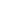) for simply supported beam subjected to concentrated loads at the midspan is computed at the end of externally bonded FRP sheet using the following equations:: is the shear stress at the end of the NSM FRP sheet.: is the normal stress at the end of the NSM FRP sheet tf: is the thickness of the NSM FRP sheet. n: is the modular ratio between concrete FRP and concrete. P: is the concentrated failure load. lo: is the unbonded length of the sheet yeff: is the distance from the neutral axis of the beam to the NSM FRP sheet. leff: is the effective moment of inertia of enhanced element. Ga: is the shear modulus of the binder agent. ta: is the thickness of the adhesive materials Ef: is the elastic modulus of the FRP sheet. Ec: is the concrete modulus of elasticity Ea: is the modulus of elasticity of adhesive material If: is the moment of inertia of FRP sheet bf: is the width of FRP sheet Ma: is the applied moment on the strengthened concrete cross section at cut off points Vo: shear force in the strengthened concrete beam at the FRP sheet cut off point The combination of both shear and normal stresses at cut off point of FRP sheet may be employed for the determination of externally bonded FRP sheet delamination. The relationship between shear and normal stresses in terms of concrete compressive and tensile strength may be expressed as: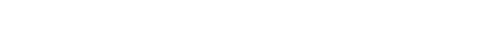Read More: Strengthening of RCC Beams in Shear using Externally Bonded FRP Plates or Strips